# 招商银行信用卡卡号识别项目（第一篇），Python OpenCV 图像处理取经之旅第 53 篇Python OpenCV 365 天学习计划，与橡皮擦一起进入图像领域吧。本篇博客是这个系列的第 53 篇。

## 学在前面## 提取卡片相关数字import cv2 as cvimport numpy as np# 读取灰度图src = cv.imread("./ka1.jpg", 0)# 寻找卡号目标区域roi = src[142:168, 42:360]# cv.imshow("roi",roi)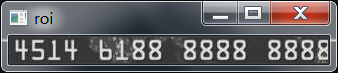# 寻找卡号目标区域roi = src[142:168, 46:364]cv.imshow("roi",roi)# 模糊卷积操作blur_roi = cv.blur(roi,(5,5))cv.imshow("blur_roi",blur_roi)med_roi = cv.medianBlur(roi,5)cv.imshow("med_roi",med_roi)gau_roi = cv.GaussianBlur(roi,(7,7),0)cv.imshow("gau_roi",gau_roi)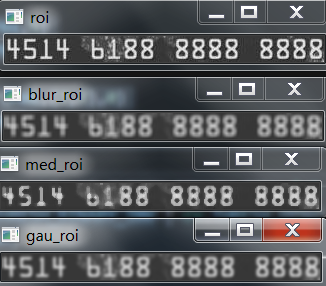# 对目标区域进行二值化操作ret, thresh = cv.threshold(    gau_roi, 0, 255, cv.THRESH_BINARY | cv.THRESH_OTSU)cv.imshow("thresh", thresh)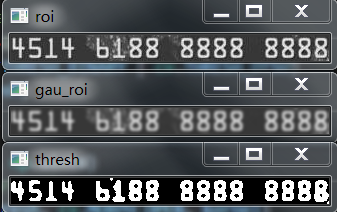# 腐蚀kernel = np.ones((3, 4), np.uint8)dst = cv.erode(thresh, kernel=kernel)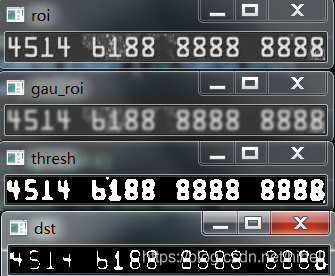# 腐蚀kernel = np.ones((3, 4), np.uint8)erode_dst = cv.erode(thresh, kernel=kernel)cv.imshow("erode_dst", erode_dst)# 膨胀kernel = np.ones((4, 4), np.uint8)dilate_dst = cv.dilate(erode_dst, kernel=kernel)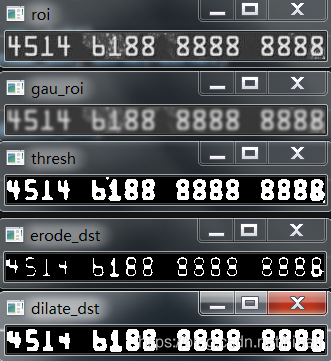# 检测外轮廓# 只检测外轮廓contours, hierarchy = cv.findContours(dilate_dst, cv.RETR_EXTERNAL,  cv.CHAIN_APPROX_SIMPLE)print(len(contours))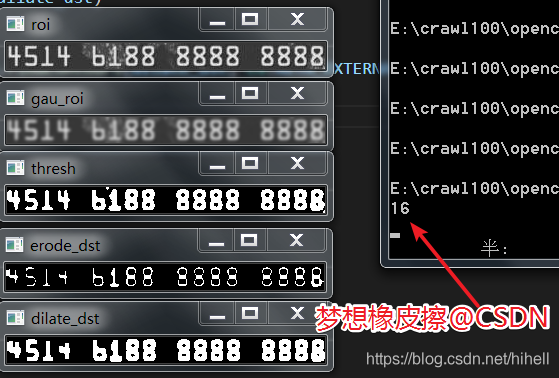target_list = []# 轮廓判断for cnt in contours:    # 轮廓面积    area = cv.contourArea(cnt)    print(area)    if area > 79:        target_list.append(cnt)print(len(target_list))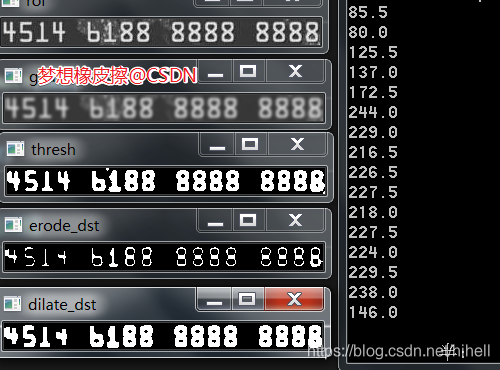for index, cnt in enumerate(target_list):    # 外接矩形    x, y, w, h = cv.boundingRect(cnt)    # 在二值化的图像上进行目标区域获取    c_roi = roi[y:y+h, x:x+w]    # 调整图像大小    # big_roi = cv.resize(c_roi, dsize=(0, 0), fx=2, fy=2)    # big_roi = cv.resize(c_roi, dsize=(0, 0))    cv.imshow("big_roi"+str(index), c_roi)    # cv.imwrite(f"./numbers/ka_{index}.png", big_roi)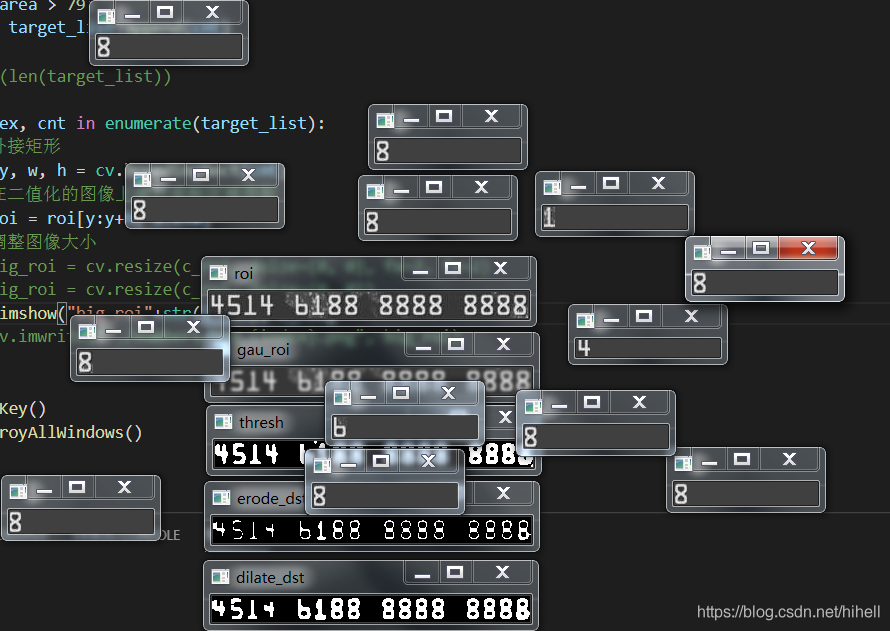big_roi = cv.resize(c_roi, dsize=(42, 66))# cv.imshow("c_roi"+str(index), c_roi)cv.imwrite(f"./numbers/ka1_{index}.png", big_roi)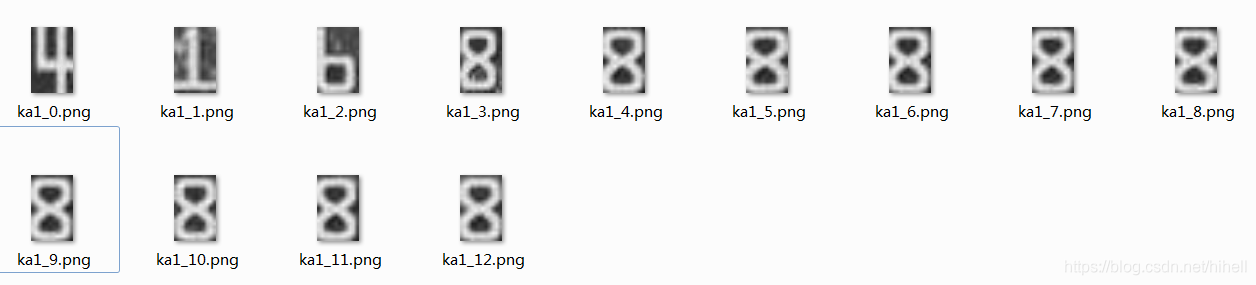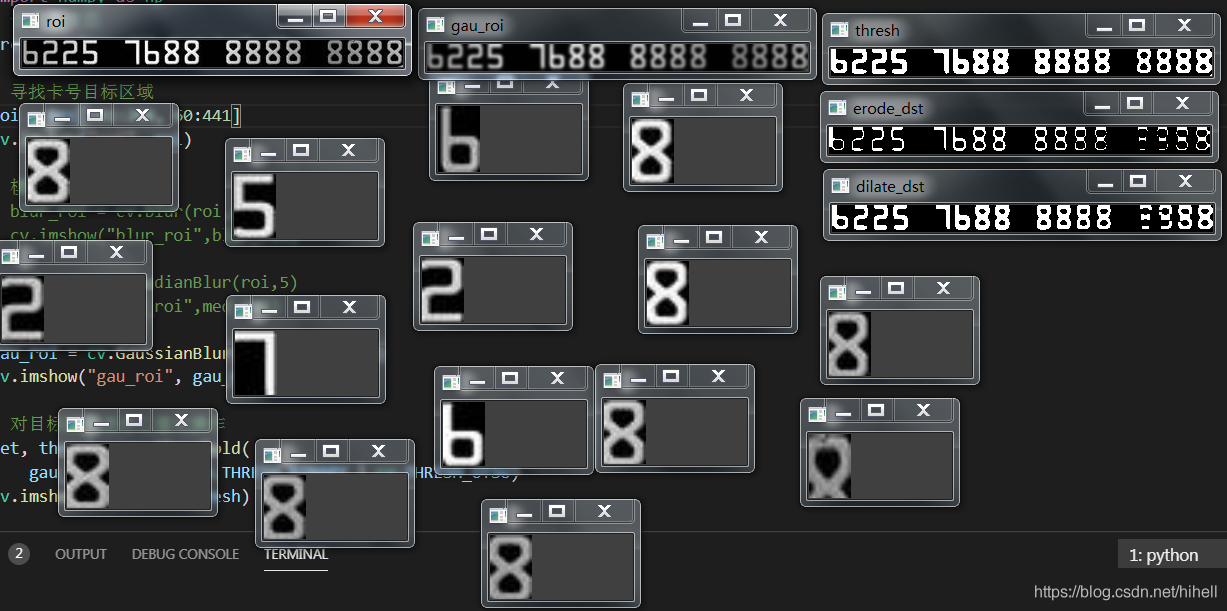import cv2 as cvimport osdef walk_file(file):    for root, dirs, files in os.walk(file):        for f in files:            file = os.path.join(root, f)            # 读取灰度图            basename = os.path.basename(file)            filename = basename.split(".")            src = cv.imread(file, 0)            ret, thresh = cv.threshold(src, 0, 255, cv.THRESH_BINARY | cv.THRESH_OTSU)            # cv.imshow("thresh", thresh)            cv.imwrite(f"./numbers/001_{filename}_bit.png", thresh)            cv.waitKey()            cv.destroyAllWindows()if __name__ == "__main__":    walk_file("./numbers")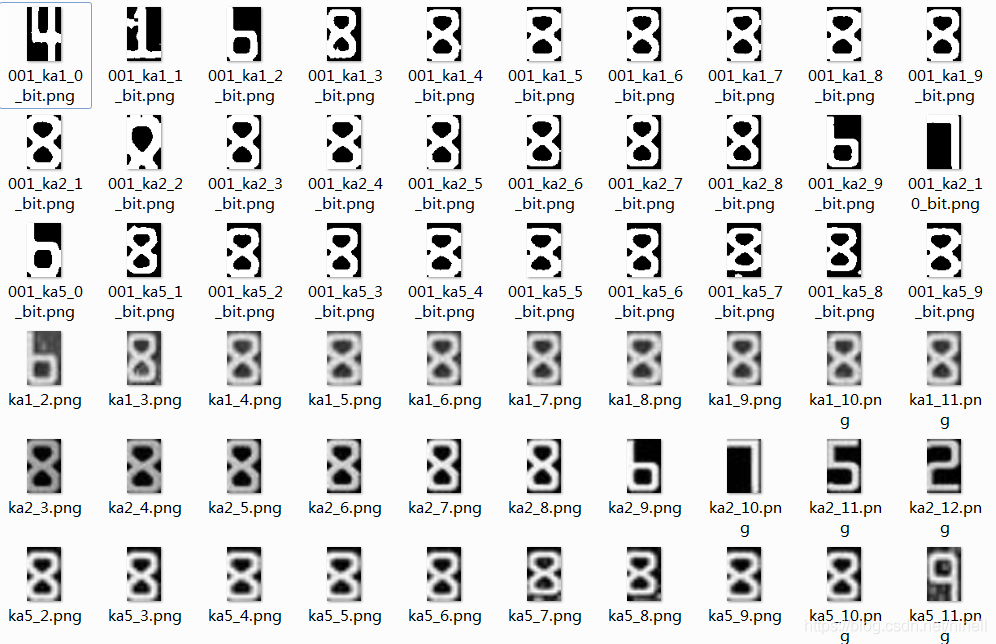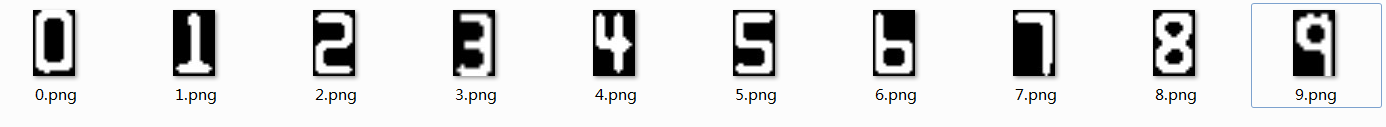## 橡皮擦的小节### 梦想橡皮擦

6 年产品经理+教学经验，3 年互联网项目管理经验； 互联网资深爱好者； 沉迷各种技术无法自拔，导致年龄被困在 25 岁； CSDN 爬虫 100 例作者。 个人公众号“梦想橡皮擦”。

## 评论